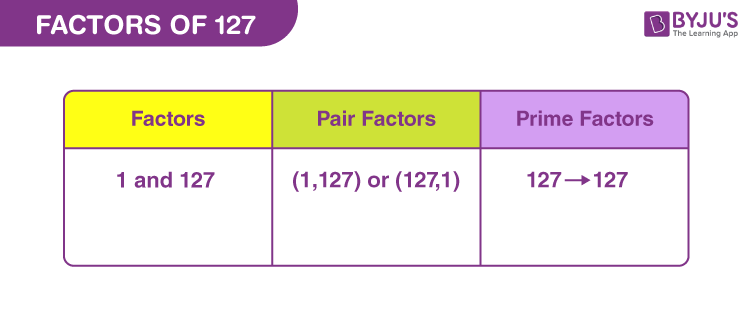# Factors of 127

In Mathematics, factors of 127 are the natural numbers by which the original number can be uniformly divided. Factors break the number into equal parts. Factors are also called the divisors of the original number.

127 is an odd number. Let us see how we can find the factors of 127 along with pair factors. Also, we will learn here the prime factorisation of 127.## How to Find the Factors of 127?

The factors of 127 should divide it evenly. Hence, after division there should be no remainder left. Also we can say, if the multiplication of two natural numbers results in 127, then these are the required factors.

Thus, we can find the factors of 127 either by dividing it by natural numbers or by multiplying two such numbers.

 127 ÷ 1 = 127 [Remainder = 0] 127 ÷ 127 = 1 [Remainder = 0]

Therefore, there are only two factors of 127. They are 1 and 127. Thus, we can conclude that 127 is a prime number (as per definition of prime numbers)

Now, if we divide 127 by any other number, then the remainder is not equal to zero.

127 ÷ 2 = 63.5

127 ÷ 7 = 18.1428571429

127 ÷ 25 = 5.08

127 ÷ 50 = 2.54

Hence, we are not able to find any other factors here.

## Pair Factors of 127

The pair factors of any natural number are the numbers in pairs, whose product results in the original number. Since 127 has only two factors, therefore the only possible pair factors are:

• 1 × 127 = 127
• 127 × 1 = 127

Therefore, the pair factors of 127 are (1, 127) or (127, 1).

Let us see, if we can consider it for negative integers.

• -1 × -127 = 127
• -127 × -1 = 127

Therefore, the negative pair factors are (-1, -127) or (-127, -1).

## Prime Factorisation of 127

Prime factorisation of any number results in prime numbers that can divide the original number, evenly. Since 127 is itself a prime number, therefore no other prime factors can divide it.

127 ÷ 127 = 1

Therefore, the prime factor of 127 is 127 itself.

 Prime factorisation of 127 = 127 Exponential form = 1271

## Solved Examples

Q.1: What is the sum of all the factors of 127?

Solution: The factors of 127 are 1 and 127.

Sum = 1 + 127 = 128

Therefore, the required sum is 128.

Q.2: What are the GCF of 127 and 137?

Solution: We have to list the factors of 127 and 137.

127 → 1, 127

137 → 1, 137

Therefore, the GCF of 127 and 73 is 1.

Q.3: In how many parts can 127 be divided equally?

Solution: 127 is a prime number and it has only two factors, i.e. 1 and 127. Therefore, we can divide 127 into 127 equal parts such that each part has 1 unit.

### Practice Questions

1. Find the GCF of 127 and 133.
2. What are the common factors of 127 and 199?
3. List down five more prime numbers after 127.
4. Is 127 divisible by 7?

## Frequently Asked Questions on Factors of 127

### What are the factors of 127?

The two factors of 127 are 1 and 127.

### Is 127 a prime number?

Yes, 127 is a prime number.

### What are the multiples of 127?

The first ten multiples of 127 are 127, 254, 381, 508, 635, 762, 889, 1016, 1143 and 1270.

### What are the common factors of 127 and 99?

The factors of 127 are 1 and 127. The factors of 99 are 1, 3, 9, 11, 33 and 99. Therefore, the common factor of 127 and 99 is 1.

### Is 127 a perfect square?

No, 127 is not a perfect square. We cannot find the square of any such natural number that could result in 127.